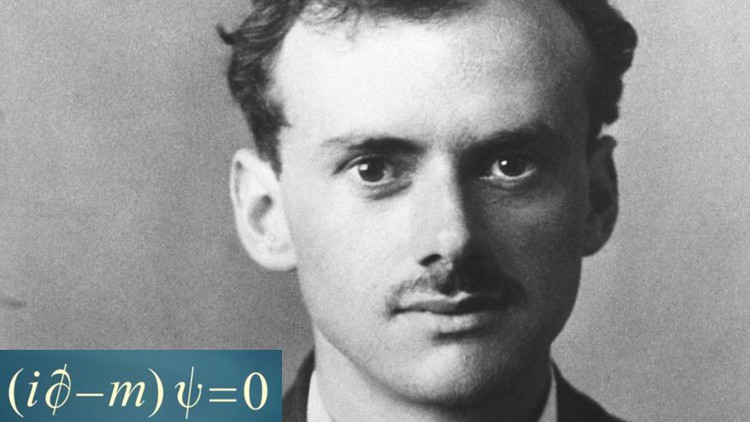# Math Intuition for Quantum Mechanics & Quantum Field TheoryQuantum Mechanics and Quantum Field Theory

What you will learn

### Anomalous magnetic moment

Description

This course aims to mathematically motivate both Quantum Mechanics (QM) and Quantum field Theory (QFT). The first part is devoted to the most important concepts and equations of QM, whereas the second part deals with QFT.

Due to the conceptual and mathematical difficulty of these subjects, some prerequisites to this course are unavoidably required. The student should be familiar with:

1) the Fourier Series and Transform;

2) Multivariable Calculus;

3) Probability theory and random variables;

4)  Classical Physics;

5) Complex Calculus (especially residues and calculation of integrals on a contour), although this is necessary only for some parts of the course devoted to QFT;

6) Special Relativity and tensors for QFT.

Note 1: the first few prerequisites might be enough if you are interested only in the first part of the course, which is related to QM (consider that this course has tens of hours’ worth of material, you might be interested only in some parts);

Note 2: I’m more than willing to reply if you have doubts/need clarifications, or -why not- have any recommendations to improve the quality of the course.

Note 3: I’ll still keep editing the videos (for example by adding notes) to make the video-lectures as clear as possible.

The references for the part on QFT are the following:

– Quantum Field Theory, M.Srednicki

– Quantum Field Theory, Itzykson & Zuber

– QFT by Mandl & Shaw

– QFT in a nutshell, A.Zee

– QFT by Ryder, Ramand

– The Quantum Theory of Fields, S.Weinberg

– Gauge Theories in Particle Physics, Aitchison & Z.Hey

English
language

Content

### Operators in Quantum Mechanics

Derivation of the energy operator in QM
Derivation of the momentum operator in QM

### Schrodinger equation, States, Eigenstates, Hermitian operators

Construction of the Schrodinger equation in QM
Eigenstates and normalized states
Importance of Hermitian operators

### Commutator, Uncertainty principle, Unitary operators

Commutator and uncertainty principle
Uncertainty principle for position and momentum
Unitary operators and time evolution of a system

### The need for Quantum Field theory, review of Classical field theory

Reconciling Quantum Mechanics with Special Relativity
Review of Classical Field Theory part 1
Review of Classical Field Theory part 2

### Noether’s theorem, Complex scalar field, symmetries, Energy momentum tensor

Klein Gordon equation derived from Classical field theory
Noether’s theorem
Example of complex scalar field & more on Noether’s theorem
Symmetry with respect to coordinate and field transformation
Energy momentum tensor, orbital angular momentum, intrinsic angular momentum
Derivation of important properties of the infinitesimal Lorentz transformation

### Quantization of a real scalar field

Quantization of a Classical Field part 1
Quantization of a Classical Field part 2
Derivation of the spectrum of the Hamiltonian part 1
Annihilation and creation operators to determine the spectrum of the Hamiltonian
Ground state and construction of states with a certain number of quanta
Definition of the number operator
Causality of space-like events part 1
Causality of space-like events part 2
Causality of space-like events part 3, an important property of Dirac delta
Causality of space-like events part 4
Green function of the Klein Gordon field part 1
Green function of the Klein Gordon field part 2
Vacuum expectation, Propagator, Time Ordering
Complex Klein Gordon field

### Interactions in Quantum Field Theory

Interacting Classical Field theory part 1
Interacting Classical Field Theory part 2
Heisenberg’s picture and Schrodinger’s picture
Interaction picture
Interacting field theory part 1
Interacting field theory part 2
Interacting Field theory, S matrix
Rewriting time ordering in the S matrix part 1, proof of Hausdorff formula
Rewriting time ordering in the S matrix part 2
Relation between Normal and Time ordered products
Wick’s theorem

### Interactions: Scattering cross section, LSZ formula

Scattering cross section part 1
Scattering cross section part 2
Scattering cross section part 3
LSZ formula part 1
LSZ formula part 2
LSZ formula part 3
LSZ formula part 4
LSZ formula part 5
LSZ formula part 6
LSZ formula part 7

### Quantization of the Electromagnetic Field

Quantization of the E.M. field part 1
Quantization of the E.M. field part 2
Quantization of the E.M. field part 3
Quantization of the E.M. field part 4
Quantization of the E.M. field part 5
Quantization of the E.M. field part 6

### Spin in Quantum Mechanics and Quantum Field Theory

Stern-Gerlach experiment
Intrinsic Angular Momentum in QM (spin) part 1
Intrinsic Angular Momentum in QM (spin) part 2
Pauli matrices, spinors
Unitary operators, Lorentz transformations, angular momenta in QFT part 1
Unitary operators, Lorentz transformations, angular momenta in QFT part 2
Unitary operators, Lorentz transformations, angular momenta in QFT part 3
Unitary operators, Lorentz transformations, angular momenta in QFT part 4
Commutator between a scalar field and angular momentum
Commutator between a vector field and angular momentum

### Fermion quantization, Dirac equation

Spinors in 4 dimensions part 1
Spinors in 4 dimensions part 2
Spinors in 4 dimensions part 3
Invariant quantities and Fermion fields
Derivation of the Dirac equation
Hamiltonian density and conserved quantities for the Dirac field
Construction of solutions of the Dirac equation part 1
Construction of solutions of the Dirac equation part 2
Quantization of the Fermion field
Important properties of the Fermion field part 1
Important properties of the Fermion field part 2, charge operator
Parity operator
Spin of the Dirac field part 1
Spin of the Dirac field part 2

### Interaction between the Dirac field and the E.M. field

Interaction of Dirac field and E.M. field
S matrix elements, interacting particles, diagrams
Amplitudes and diagrams of processes
Why 1st order terms in the S matrix do not give contribution
Calculation of amplitudes of 2nd order processes
Feynman Rules part 1
Feynman Rules part 2